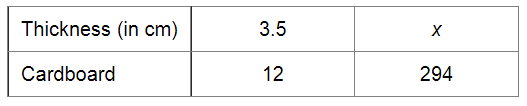# If the thickness of a pile of 12 cardboards is 35 mm,`
Question:

If the thickness of a pile of 12 cardboards is 35 mm, find the thickness of a pile of 294 cardboards.

Solution:

Let x cm be the thickness of a pile of 294 cardboards.Since the pile of the cardboards and its thickness are in direct variation, we have:

$\frac{3.5}{x}=\frac{12}{294}$

$\Rightarrow 3.5 \times 294=x \times 12$

$\Rightarrow x=\frac{3.5 \times 294}{12}$

$=\frac{1029}{12}$

$=85.75 \mathrm{~cm}$

Thus, the thickness of a pile of 294 cardboards will be $85.75 \mathrm{~cm}($ or $857.5 \mathrm{~mm})$.